Courses

# Motion in One Dimension(Part- 1) - Physics, Solution by DC Pandey NEET Notes | EduRev

## JEE : Motion in One Dimension(Part- 1) - Physics, Solution by DC Pandey NEET Notes | EduRev

The document Motion in One Dimension(Part- 1) - Physics, Solution by DC Pandey NEET Notes | EduRev is a part of the JEE Course Physics Class 11.
All you need of JEE at this link: JEE

Introductory Exercise 3.1

Q.1. When a particle moves with constant velocity its average velocity, its instantaneous velocity and its speed all are equal. Is this statement true or false?
Ans. True

Suppose a particle is moving with constant velocity  v (along the axis of x).
Displacement of particle in time t1 = vt1
Displacement of particle in time t2 = vt2
∴ Displacement of the particle in the time interval Δt (= t2 - t1)
= vt2 - vt1
= v (t2 - t1)
∴ Average velocity in the time interval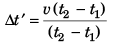= v
Now, as the particle is moving with constant velocity (i.e., with constant speed in a given direction) its velocity and speed at any instant will obviously be v.

Ques 2: A stone is released from an elevator going up with an acceleration of g/2. What is the acceleration of the stone just after release?
Ans. g (downwards)

As the stone would be free to acceleration under earth’s gravity it acceleration will be g.

Q.3. A dock has its second hand 2.0 cm long. Find the average speed and modulus of average velocity of the tip of the second hand in 15 s.
Ans.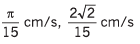A second hand takes 1 min i.e., 60s to complete one rotation (i.e., rotation by an angle of 2π rad).
∴ Angular speed of second hand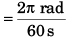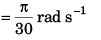Linear speed of its tip = radius x angular speed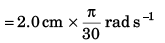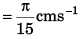As the tip would be moving with constant speed.
Average speed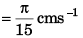In 15 s the second hand would rotate through 90° i.e., the displacement of its tip will be r√2.
∴ Modulus of average velocity of the tip of second hand in 15 s.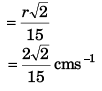Q.4. (a) Is it possible to be accelerating if you are travelling at constant speed?
(b) Is it possible to move on a curved path with zero acceleration, constant acceleration, variable acceleration?
Ans. (a) Yes, in a uniform circular motion

(b) No, yes (projectile motion), yes

(a) Yes. By changing direction of motion, there will be change in velocity and so acceleration.
(b) (i) No. In curved path there will always be acceleration. (As explained in the previous answer no. 3)
(ii) Yes. In projectile motion the path of the particle is a curved one while acceleration of the particle remains constant.
(iii) Yes. In curved path the acceleration will always be there. Even if the path is circular with constant speed the direction of the acceleration of the particle would every time be changing.

Q.5. A particle is moving in a circle of radius 4 cm with a constant speed of 1 cm/s.
Find:
(a) Time period of the particle.
(b) Average speed, average velocity, and average acceleration in a time interval from t = 0 to t = T/4. Here, T is the time period of the particle. Give only their magnitudes.
Ans. (a) 25 .13 s

(b) 1 cm/s , 0 .9 cm/s , 0 .23 cm/s2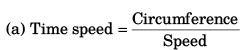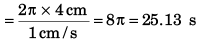(b) As particle is moving with constant speed of 1 cm/s, its average speed in any time interval will be 1 cm/s.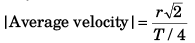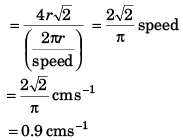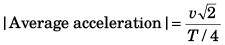(where v = speed)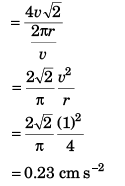Q.6. A particle moves in a straight line with constant speed of 4 m/s for 2 s, then with 6 m/s for 3 s. Find the average speed of the particle in the given time interval.
Ans. 5.2 m/s

Distance = Speed x time
D1 = v1t1
D2 = v2 t2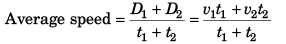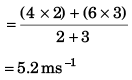Offer running on EduRev: Apply code STAYHOME200 to get INR 200 off on our premium plan EduRev Infinity!

## Physics Class 11

73 videos|313 docs|177 tests

,

,

,

,

,

,

,

,

,

,

,

,

,

,

,

,

,

,

,

,

,

,

,

,

;